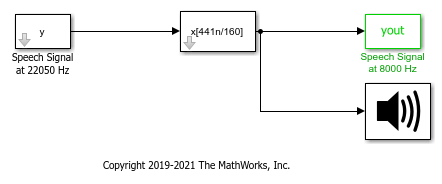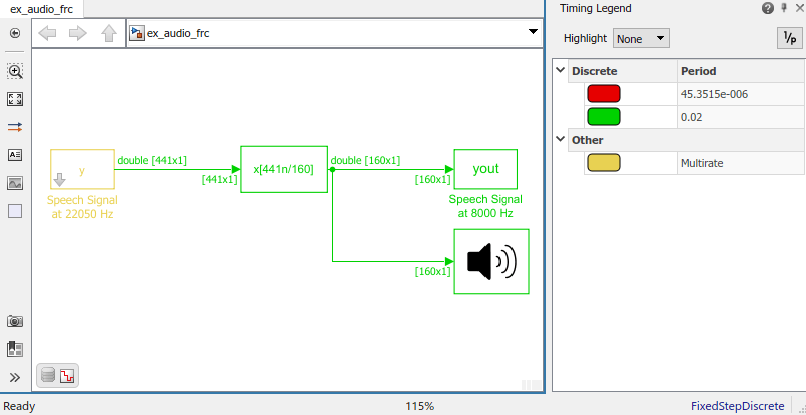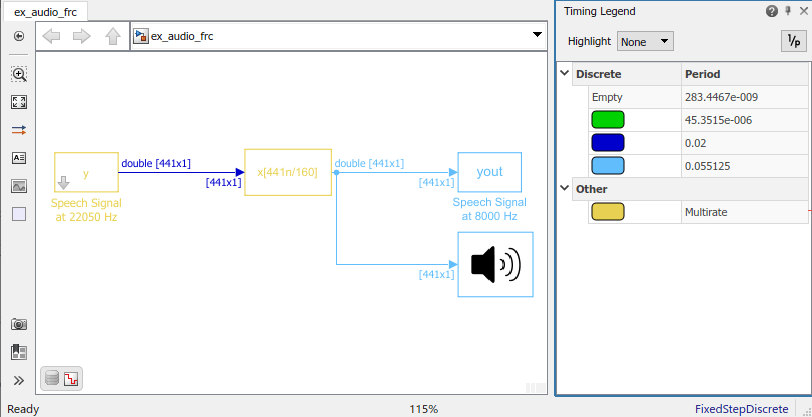# Convert Sample Rate of Speech Signal

This example shows how to use the FIR Rate Conversion block to convert the sample rate of a speech signal. It compares the output/signal behavior in two scenarios -single rate processing and multirate processing. Convert the sample rate of a speech signal from 22.5 kHz to 8 kHz.

### Enforce single-rate processing

The output signal rate and the input signal rate in Simulink® are the same.

The interpolation factor L is set to 160 and the decimation factor K is set to 441. The output frame size is L/K times the input frame size.

Open and run the model to listen to the output. High frequency content has been removed from the signal, although the speech sounds basically the same.The output and the input signal rate are the same in Simulink. This is shown by the green color-coded signal lines at the input and output of the block.### Allow multirate processing

Change the Rate options parameter to `Allow multirate processing`.

Run the model. You can see that the output signal and the blocks connected to the output signal operate at a rate that is L/K times the rate at which the input signal operates in Simulink.